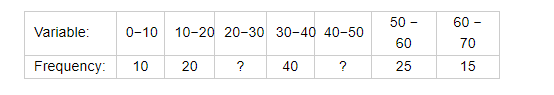# An incomplete distribution is given as follows:

Question:

An incomplete distribution is given as follows:You are given that the median value is 35 and the sum of all the frequencies is 170. Using the median formula, fill up the missing frequencies.

Solution:

Let the frequency of the class 20-30 be $f_{1}$ and that of class 40-50 be $f_{2}$. The total frequency is 170. So,

$10+20+f_{1}+40+f_{2}+25+15=170$

So, $f_{1}+f_{2}=60$.....(1)

It is given that median is 35 which lies in the class 30-40. So 30-40 is the median class.

Now, lower limit of median class $(l)=30$

Height of the class $(h)=10$

Frequency of median class $(f)=40$

Cumulative frequency of preceding median class $(F)=10+20+f_{1}$

Total frequency $(N)=170$

Formula to be used to calculate median,

$=l+\left(\frac{\frac{N}{2}-F}{f}\right)(h)$

Where,

I - Lower limit of median class

$h$ - Height of the class

$f$ - Frequency of median class

$F$ - Cumulative frequency of preceding median class

$N$ - Total frequency

Put the values in the above,

$35=30+\left(\frac{\frac{170}{2}-\left(30+f_{1}\right)}{40}\right)(10)$

$f_{1}=-20+\frac{170}{2}-30$

$=35$

Using equation (1), we have

$f_{2}=25$

Therefore,

$f_{1}=35$

$f_{2}=25$